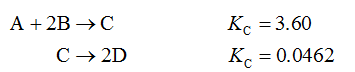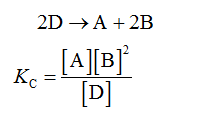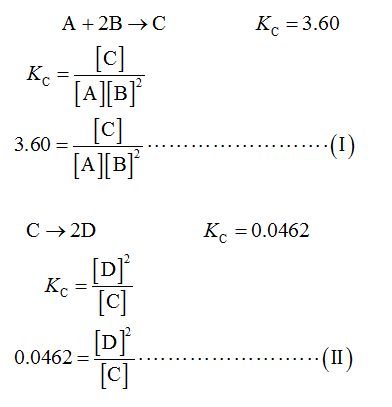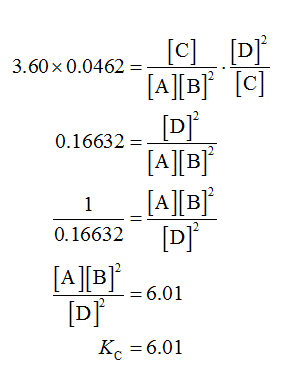# Calculate the value of the equilibrium constant Kc for the reaction2D=A+2Bfrom the following information:A+2B=CKc=3.60C=2DKc=0.0462

Question
2 views

Calculate the value of the equilibrium constant Kc for the reaction
2D=A+2B

from the following information:

A+2B=C

Kc=3.60

C=2D

Kc=0.0462

check_circle

star
star
star
star
star
1 Rating
Given Data:Calculations:

The equilibrium constant (Kc) for the given reaction is shown below.The given reactions-Multiply equation (I) and equation (II).Therefore, the equilibrium constant (Kc) for the given reaction is 6.01.

...

### Want to see the full answer?

See Solution

#### Want to see this answer and more?

Solutions are written by subject experts who are available 24/7. Questions are typically answered within 1 hour.*

See Solution
*Response times may vary by subject and question.
Tagged in